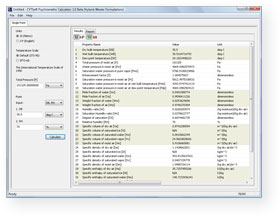﻿ CYTSoft Psychrometric Calculator
 CYTSoft Psychrometric Calculator 1.0

#### OverviewCYTSoft Psychrometric Calculator 1.0 provides engineers and scientists with highly accurate thermodynamic properties of moist air. It calculates as many as 42 properties completely based on ASHRAE's Hyland-Wexler Formulations, which treat dry air, water vapor and moist air as real gases, rather than ideal gases.
• Platform: Windows 98/2000 or higher
• Limitations: 30-day trial
• File Size: 0.9 MB
• Current Version: 1.0
• Release Date: November 11, 2008
• Units: SI and I-P

#### Range of validity

• Pressure: 1 Pa to 5 MPa
• Dry-bulb temperature: -50 to 200 deg C
• Humidity ratio: 0 to 100 000 (kg moisture)/(kg dry air), or 0 to 100 000 000 (g moisture)/(kg dry air)

#### Equation of state

Moist air is treated as mixture of two real gases. The P-v-T equation for each component and mixture is described by a virial equation of state

Pv/RT = Z = 1 + B/v + C/v2 + ...

where

P = pressure
v = molar specific volume
T = absolute temperature
R = gas constant
Z = compressibility factor
B = second virial coefficient
C = third virial coefficient

Fourth and higher-order virial coefficients are assumed to be negligible. The virial coefficients are functions of temperature, and they account for the deviation of actual gases from ideal gases.

#### Input

You can select any one of the following 24 combinations of input variables for each calculation:

1. DB, RH, P
2. DB, WB, P
3. DB, DP, P
4. DB, W, P
5. DB, h, P
6. DB, s, P
7. DB, v, P
8. WB, DP, P
9. WB, RH, P
10. WB, W, P
11. WB, h, P
12. WB, s, P
13. WB, v, P
14. DP, RH, P
15. DP, h, P
16. DP, s, P
17. DP, v, P
18. RH, W, P
19. RH, h, P
20. RH, s, P
21. RH, v, P
22. W, h, P
23. W, s, P
24. W, v, P

where

DB = dry bulb temperature of moist air
WB = wet bulb temperature of moist air
DP = dew point temperature of moist air
RH = relative humidity of moist air
W = humidity ratio of moist air
h = specific enthalpy of moist air
s = specific entropy of moist air
v = specific volume of moist air
P = total pressure

#### Output

1. Dry bulb temperature
2. Wet bulb temperature
3. Dew point temperature
4. Total pressure of moist air
5. Water pressure in moist air
6. Saturation water pressure in pure vapor
7. Enhancement factor
8. Saturation water pressure in moist air
9. Saturation water pressure in moist air at wet bulb temperature
10. Saturation water pressure in moist air at dew point temperature
11. Mole fraction of water
12. Mole fraction of air
13. Weight fraction of water
14. Weight fraction of air
15. Humidity ratio
16. Saturation humidity ratio
17. Degree of saturation
18. Relative humidity
19. Absolute humidity
20. Specific volume of dry air
21. Specific volume of saturated ice
22. Specific volume of saturated water
23. Specific volume of saturated vapor
24. Specific volume of moist air
25. Specific density of dry air
26. Specific density of saturated ice
27. Specific density of saturated water
28. Specific density of saturated vapor
29. Specific density of moist air
30. Specific enthalpy of dry air
31. Specific enthalpy of saturated ice
32. Specific enthalpy of saturated water
33. Specific enthalpy of saturated vapor
34. Specific enthalpy of moist air
35. Specific entropy of dry air
36. Specific entropy of saturated ice
37. Specific entropy of saturated water
38. Specific entropy of saturated vapor
39. Specific entropy of moist air
40. Compressibility factor for dry air
41. Compressibility factor for saturated vapor
42. Compressibility factor for moist air

#### References

1. ASHRAE. 2005. 2005 ASHRAE Handbook - Fundamentals. ASHRAE.
2. Hyland, R.W. and A. Wexler. 1983a. Formulations for the thermodynamic properties of dry air from 173.15 K to 473.15 K, and of saturated moist air from 173.15 K to 372.15 K, at pressures to 5 MPa. ASHRAE Transactions 89(2A):520-535.
3. Hyland, R.W. and A. Wexler. 1983b. Formulations for the thermodynamic properties of the saturated phases of H2O from 173.15 K to 473.15 K. ASHRAE Transactions 89(2A):500-519.
4. Olivieri, J. 1996. Psychrometrics - Theory and practice. ASHRAE.
5. Preston-Thomas, H. 1990. The international temperature scale of 1990 (ITS-90). Metrologia 27(1):3-10.